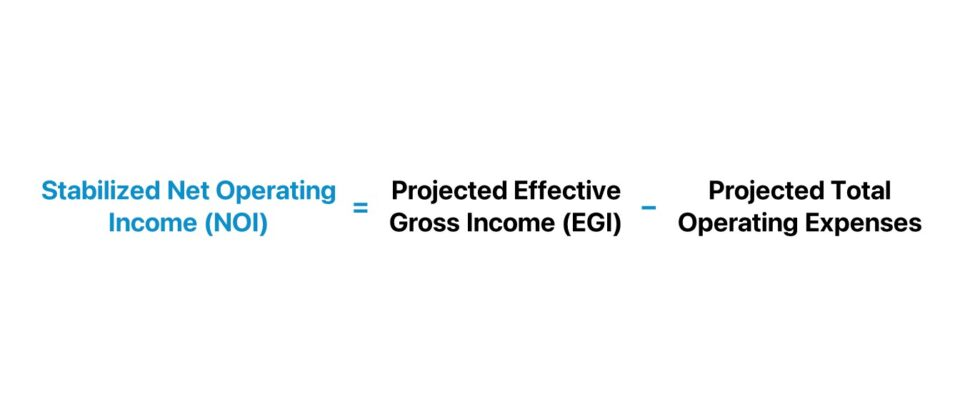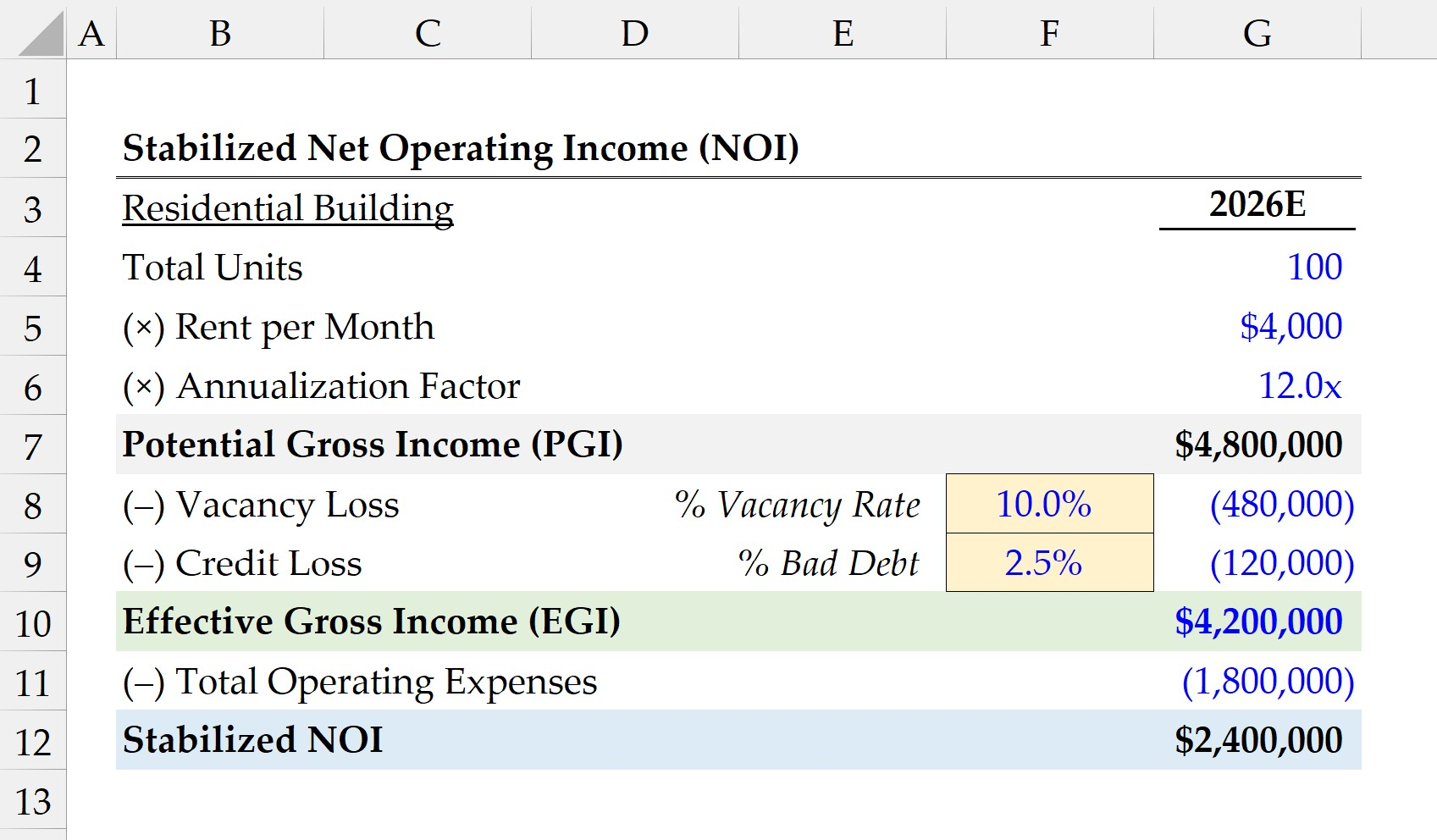Welcome to Wall Street Prep! Use code at checkout for 15% off.# Stabilized NOI

Guide to Understanding Stabilized Net Operating Income (NOI)## What is the Definition of Stabilized NOI?

The stabilized NOI, a common real estate investing metric, reflects the projected net operating income (NOI) of a property investment upon reaching a “steady state” level of operations.

• Stabilization → The term “stabilization” describes the point in time when a property is fully operational, either in proximity or at its profit potential. Thus, stabilized properties are property investments in which the necessary (or discretionary) construction, repair, and renovation work is complete.
• Occupancy – The other component in the criteria is the occupancy rate, where the stabilized property must exhibit an occupancy rate – i.e. the inverse of the vacancy rate – indicative of the occupancy once the property is no longer under construction and fully operational.

Since the real estate property is operating near capacity and capable of generating income, the stabilized NOI is a forward-looking measure of profitability.

Understanding the profit potential of a given property upon reaching “stabilization” is critical to estimating the implied yield on a potential investment.

The approximate debt capacity of the property can also be determined using the stabilized NOI, i.e. the maximum debt burden that the property can handle before the risk of default is at an unmanageable level.

In particular, the commercial real estate (CRE) market tends to pay close attention to stabilized metrics because of unpredictable internal and external market factors, such as the vacancy rate, tenant turnover, and economic conditions, which can cause the performance of commercial properties to fluctuate substantially.

Further, the lack of stability in income is counterintuitive to real estate projects funded using leverage, i.e. debt financing, which is more prevalent in the CRE market.

• Initial NOI → On the initial purchase date of a commercial property, the NOI is often lower and can require years before investment to start producing sufficient income.
• Stabilized NOI → Once the property reaches a steady state, the property’s NOI is deemed to be “stabilized” and is now a more accurate profit metric to determine the value of a property (and potential yield).## How to Calculate Stabilized NOI?

Calculating a property’s stabilized net operating income (NOI) consists of estimating the potential gross income (PGI), deducting vacancy and credit losses, and subtracting operating expenses.

1. Potential Gross Income (PGI) → The potential gross income (PGI), or gross potential income (GPI), is the maximum amount of income that a property could generate if operating at full capacity, i.e. 100% occupancy rate and no issues with collection of rent payments.
2. Vacancy and Collection Losses → The losses attributable to vacancy and credit (collection) issues refer to the income lost from vacant units or the inability to collect rent from tenants.
3. Effective Gross Income (EGI) → The effective gross income (EGI) is the remaining income after deducting the potential gross income (PGI) from the vacancy and collection losses.
4. Total Operating Expenses → The operating expenses section comprises of the costs associated with running the day-to-day operations of the property, such as property management fees, property insurance, repairs, property taxes, and so forth.
5. Net Operating Income (NOI) → The net operating income (NOI) is calculated by subtracting the effective gross income (EGI) from the total operating expenses expected to be incurred.

Note: The stabilized NOI is a forward-looking, normalized metric, so adjustments are necessary to remove the effects of any non-recurring items.

## Net Operating Income (NOI) Formula

The formula to calculate the net operating income (NOI) is the difference between the effective gross income (EGI) and operating expenses.

Net Operating Income (NOI) = Effective Gross Income (EGI) Total Operating Expenses

Where:

• Potential Gross Income (PGI) = Monthly Rent per Unit × Total Number of Units × 12 Months
• Vacancy Loss = Potential Gross Income (PGI) × Vacancy Rate (% of PGI)
• Credit Loss = Potential Gross Income (PGI) × Bad Debt (% of PGI)
• Effective Gross Income (EGI) = Potential Gross Income (PGI) – Vacancy Loss – Credit Loss

Because the net operating income (NOI) metric is computed on a stabilized basis, a critical step is to ensure the figures used in the formula – including the costs – are all on a normalized basis (and are “scrubbed” for non-recurring items).

The sum of the operating expenses should be inclusive of all annual operating costs, such as the following:

• Routine Maintenance Costs
• Property Management Fees
• Property Insurance
• Property Taxes
• Inspection Fees

The stabilized net operating income (NOI) concept is the difference between the property’s effective gross income (EGI) and total operating expenses.

## Stabilized NOI Formula

The formula to calculate the stabilized NOI is identical to the standard net operating income (NOI).

However, the distinction is that the income and expense figures used here must be representative of the property’s performance at stabilization.

Stabilized NOI = Projected Effective Gross Income (EGI)  Projected Total Operating Expenses

Again, the figures must reflect the normalized operating performance of the property investment, with adjustments to remove any non-operating or non-recurring items.

The stabilized NOI is an input in the formula to calculate the cap rate, which reflects the rate of return expected by a real estate investor on an investment property.

Cap Rate (%) = Stabilized NOI ÷ Property Value

The reason the stabilized NOI is used in the cap rate formula is to ensure that the income component of the metric is on a normalized basis, i.e. the property is fully functional and producing income on behalf of the property owner.

## Stabilized NOI Calculator

We’ll now move to a modeling exercise, which you can access by filling out the form below.#### Get the Excel Template!Submitting...

## 1. Real Estate Property Investment Assumptions

Suppose you’re a real estate investor tasked with calculating the stabilized NOI of a property investment currently under construction and repair work.

The real estate development project – a residential building with a total of 100 units – is expected to be complete by 2026.

Once the residential building is stabilized, the pro forma projections are as follows.

2026E Projections

• Total Number of Units = 100
• Rent per Month = \$4,000
• Annualization Factor = 12.0x
• Vacancy Loss (% of PGI) = 10.0%
• Credit Loss (% of PGI) = 2.5%

The first step is to calculate the potential gross income (PGI) by multiplying the total number of units available for rent by the rent per month, followed by converting the monthly PGI into an annualized PGI.

• Potential Gross Income (PGI) = 100 Units × \$4,000 × 12 = \$4,800,000

From the potential gross income (PGI), we must deduct vacancy and credit losses to calculate the effective gross income (EGI), which is \$4.2 million.

• Vacancy Loss = \$480,000
• Credit Loss = \$120,000
• Effective Gross Income (EGI) = \$4,800,000 – \$480,000 – \$120,000 = \$4,200,000

## 2. Stabilized NOI Calculation Example

In the next section, we’ll estimate the stabilized NOI of the residential building by deducting total operating expenses from the effective gross income (EGI).

For the total operating expenses incurred by the property per year, we’ll assume the annual spending was \$1.8 million.

• Total Operating Expenses = \$1,800,000

Since we have the two required inputs, the final step is to deduct the total operating expenses from the effective gross income (EGI) to arrive at an implied stabilized NOI of \$2.4 million.

• Stabilized NOI = \$4,200,000 – \$1,800,000 = \$2,400,00020+ Hours of Online Video Training

### Master Real Estate Financial Modeling

This program breaks down everything you need to build and interpret real estate finance models. Used at the world's leading real estate private equity firms and academic institutions.

Inline Feedbacks